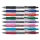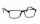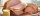# Divide 8

Divide 6840 by x y and z, in such a way that x has twice as much as y, who has half as much as z

Result

x =  2736
y =  1368
z =  2736

#### Solution:Leave us a comment of this math problem and its solution (i.e. if it is still somewhat unclear...):Be the first to comment!#### Following knowledge from mathematics are needed to solve this word math problem:

Do you have a linear equation or system of equations and looking for its solution? Or do you have quadratic equation?

## Next similar math problems:

1. Age problemsA) Alex is 3 times as old as he was 2 years ago. How old is he now? b) Casey was twice as old as his sister 3 years ago. Now he is 5 years older than his sister. How old is Casey? c) Jessica is 4 years younger than Jennifer now. In 10 years, Jessica wi
2. Forestry workersIn the forest is employed 56 laborers planting trees in nurseries. For 8 hour work day would end job in 37 days. After 16 days, 9 laborers go forth? How many days are needed to complete planting trees in nurseries by others, if they will work 10 hours a d
3. CoffeeIn stock are three kinds of branded coffee prices: I. kind......248 Kč/kg II. kind......134 Kč/kg III. kind.....270 Kč/kg Mixing these three species in the ratio 10:7:7 create a mixture. What will be the price of 1100 grams of this mixture?
4. MotionIf you go at speed 3.7 km/h, you come to the station 42 minutes after leaving the train. If you go by bike to the station at speed 27 km/h, you come to the station 56 minutes before its departure. How far is the train station?
5. Bike rideMarek rode a bike ride. In an hour, John followed him on the same route by car, at an average speed of 72 km/h, and in 20 minutes he drove him. Will he determine the length of the way that Marek took before John caught up with him, and at what speed did Ma
6. ExcavationMr. Billy calculated that excavation for a water connection dig for 12 days. His friend would take 10 days. Billy worked 3 days alone. Then his friend came to help and started on the other end. On what day since the beginning of excavation they met?
7. TV transmitterThe volume of water in the rectangular swimming pool is 6998.4 hectoliters. The promotional leaflet states that if we wanted all the pool water to flow into a regular quadrangle with a base edge equal to the average depth of the pool, the prism would have.
8. Two penTwo pen and one notebook costs \$56. One pen costs 1/5 of one notebook. How much is the one notebook?
9. Camel and water84% of the camel's weight is water. After drinking, its weight increased to 832 kg and water accounted for 85% of its weight. How much did it weigh before drinking?
10. The sum 4The sum of Robin's age is 45. Seven years ago, Robin was 16 years more than one half as old as Bruno then. How old is Bruno?
11. Faces diagonalsIf the diagonals of a cuboid are x, y, and z (wall diagonals or three faces) respectively than find the volume of a cuboid. Solve for x=1.2, y=1.7, z=1.45
12. Two chordsCalculate the length of chord AB and perpendicular chord BC to circle if AB is 4 cm from the center of the circle and BC 8 cm from the center of the circle.The fruit basket is five times heavier than the empty basket. Filled is 20kg heavier than empty. Determine the weight of the fruit in the basket.The cyclist on three-day trip travel 30% of the total route on the first day, 3/5 of the rest on the second day and 35 km on the third day. How many kilometers did travel cyclists each day and how many?If water flows into the pool by two inlets, fill the whole for 8 hours. The first inlet filled pool 6 hour longer than second. How long pool take to fill with two inlets separately?There are 36 pupils in the class. Nine girls wear glasses. Boys with glasses are five less than girls without glasses. Boys without glasses are two times more than girls without glasses. How many boys and how many girls?Casey bought a 15.4 pound turkey and an 11.6 pound ham for thanksgiving and paid \$38.51. Her friend Jane bought a 10.2 pound turkey and a 7.3 pound ham from the same store and paid \$24.84. Find the cost per pound of turkey and the cost per pound of ham.